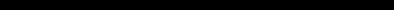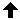# SINCLAIR ZX SPECTRUM BASIC ProgrammingCHAPTER 13
Conditions

#### Summary

AND, OR, NOT

We saw in Chapter 3 how an IF statement takes the form

IF condition THEN ...

The conditions there were the relations (=, <, >, <=, >= and <>), which compare two numbers or two strings. You can also combine several of these, using the logical operations, AND, OR and NOT.

One relation AND another relation is true whenever both relations are true, so you could have a line like:

IF a\$="yes" AND x>0 THEN PRINT x

in which x only gets printed if a\$=''yes" and x>0. The BASIC here is so close to English that it hardly seems worth spelling out the:details. As in English, you can join lots of relations together with AND, and then the whole lot is true if all the individual relations are.

One relation OR another is true whenever at least one of the two relations is true. (Remember that it is still true if both the relations are true; this is something that English doesn't always imply.)

The NOT relationship turns things upside down. The NOT relation is true whenever the relation is false, and false whenever it is true!

Logical expressions can be made with relations and AND, OR and NOT, just as numerical expressions can be made with numbers and +, - and so on; you can even put them in brackets if necessary. They have priorities in the same way as the usual operations +, -, *, / and, do: OR has the lowest priority, then AND, then NOT, then the relations, and the usual operations.

NOT is really a function, with an argument and a result, but its priority is much lower than that of other functions. Therefore its argument does not need brackets unless it contains AND or OR (or both). NOT a=b means the same as NOT (a=b) (and the same as a<>b, of course).

<> is the negation of = in the sense that it is true if, and only if, = is false. In other words,

a<>b is the same as NOT a=b
and also
NOT a<>b is the same as a=b

Persuade yourself that >= and <= are the negations of < and > respectively: thus you can always get rid of NOT from in front of a relation by changing the relation.

Also,

NOT (a first logical expression AND a second)

is the same as

NOT (the first) OR NOT (the second)

and

NOT (a first logical expression OR a second)

is the same as

NOT (the first) AND NOT (the second).

Using this you can work NOTs through brackets until eventually they are all applied to relations, and then you can get rid of them. Logically speaking, NOT is unnecessary, although you might still find that using it makes a program clearer.

The following section is quite complicated, and can be skipped by the fainthearted!

Try

PRINT 1=2,1<>2

which you might expect to give a syntax error. In fact, as far as the computer is concerned, there is no such thing as a logical value: instead it uses ordinary numbers, subject to a few rules.

 i. =, <, >, <=, >= and <> all give numeric results: 1 for true, and 0 for false. Thus the PRINT command above printed 0 for '1=2', which is false, and 1 for '1<>2', which is true. ii. In IF condition THEN ... the condition can be actually any numeric expression. If its value is 0, then it counts as false, and any other value lincluding the value of 1 that a true relation gives) counts as true. Thus the IF statement means exactly the same as IF condition <>0 THEN ... iii. AND, OR and NOT are also number-valued operations. x AND y has the value {x if y is true (non‑zero)}  {0 (false), if y is false (zero) x OR y has the value {1 (true), if y is true (non‑zero)  {x, if y is false (zero) NOT x has the value {0 (false), if x is true (non‑zero)  {1 (true), if x is false (zero) iv. (Notice that 'true' means 'non-zero' when we're checking a given value, but it means '1' when we're producing a new one.)

Read through the chapter again in the light of this revelation, making sure that it all works.

In the expressions x AND y, x OR y and NOT x, x and y will usually take the values 0 and 1 for false and true. Work out the ten different combinations (four for AND, four for OR and two for NOT) and check that they do what the chapter leads you to expect them to do.

Try this program:

10 INPUT a
20 INPUT b
30 PRINT (a AND a>=b)+(b AND a<b)
40 GO TO 10

Each time it prints the larger of the two numbers a and b. Convince yourself that you can think of

x AND y

as meaning

x if y (else the result is 0)

and of

x OR y

as meaning

x unless y (in which case the result is 1)

An expression using AND or OR like this is called a conditional expression. An example using OR could be

LET total price=price less tax*(1.15 OR v\$="zero rated")

Notice how AND tends to go with addition (because its default value is 0), and OR tends to go with multiplication (because its default value is 1).

You can also make string valued conditional expressions, but only using AND.

 x\$ AND y has the value {x\$ if y is non-zero {"" if y is zero

so it means x\$ if y (else the empty string).

Try this program, which inputs two strings and puts them in alphabetical order.

10 INPUT "type in two strings"'a\$,b\$
20 IF a\$>b\$ THEN LET c\$=a\$: LET a\$=b\$: LET b\$=c\$
30 PRINT a\$;" ";("<" AND a\$<b\$)+("=" AND a\$=b\$);" ";b\$
40 GO TO 10

#### Exercise

 1. BASIC can sometimes work along different lines from English. Consider, for instance, the English clause 'If a doesn't equal b or c'. How would you write this in BASIC? The answer is not IF A<>B OR C nor is it IF A<>B OR A<>C